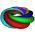## Saturday, October 6, 2012

### September Essential Questions (Final)

Ask yourself if you can answer all of these questions.  They were the gems that students asked during the first month of school that I wrote on my low-tech note card in my dress shirt pocket.

Parents, ask your students if they understand and can explain. The ones in bold are for Pre-Calc only.

My Best,

Mr. Morrissey

1.This comment has been removed by the author.

2.Hmm, some feedback:

1. I assume you mean x-intercept for a line, because there's no general method to find exact solutions to f(x) = 0 for any arbitrary function (!) So I'd reword it to "Given the function f(x) = ax + b for some fixed constants a and b, where a is not 0, show that there is a unique x-intercept and explain how to find it."

2. Assuming there's no cube root button ;) (then the easiest way would be to raise the number to the 0.33333333... or 1/3 power)

3. I'm not sure what the point of this is. If it's to demonstrate that (-a)/b = a/(-b) = -(a/b), then I guess it's okay. The intent is not very clear at the moment.

4 & 5. The question "Is 1 a prime number?" makes the question "What is the smallest prime number?" unnecessary.

6. The question is imprecise because it doesn't mention that you are talking about integers -- if you were talking about real numbers, then the answer would be different. Between 1 and 5, inclusive, there are 5 integers, infinitely many rational numbers (but countably many), and infinitely many real numbers (but uncountably many). You should attend to precision and say instead, "Explain why if a,b are integers and a < b, then the number of integers between a and b, inclusive, is precisely b - a + 1."

7. I guess that is fine.

8. I guess that is fine.

9. This really isn't a math question... It's a semantic issue. We can say "root of a function" and "zero of a function" and it means exactly the same thing (in my opinion). What they both mean is encapsulated by the following definition: "A root (or zero) of a function f is a number r in the domain of f such that f(r) = 0."

10. This is somewhat vague. What kind of answer are you expecting?

11. I'm lost on this one! Do you mean whether you can divide P(x) by (x-a) then by (x-b), or by (x-b) then by (x-a), and get the same result? (And do you see how much clearer this revised question is? ;) )

12. Common denominator of an equation isn't well-defined. Besides, in the equation x/a + y/b = 6, you just have to think of it as the following equivalent equation: (1/a)x + (1/b)y = 6; note that 1/a and 1/b are just constants and you can treat them as such. You don't have to multiply by any common denominator!

13. I would suggest the following question instead: "Why does one sometimes get extraneous solutions to an equation when following a process of solving it? For what types of equations can we be guaranteed not to get extraneous solutions?"

3.Yongyi,

These were all questions by students.

4.Ah, that explains it. I guess you can't expect students' questions to be perfectly formed! I was thinking of essential questions as questions written by the teacher to be thought about over the course of the unit.